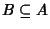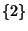## Subset

A portion of a Set.is a subset of(written) Iff every member ofis a member of. Ifis a Proper Subset of(i.e., a subset other than the set itself), this is written.

A Set ofelements hassubsets (including the set itself and the Empty Set). For sets of, 2, ... elements, the numbers of subsets are therefore 2, 4, 8, 16, 32, 64, ... (Sloane's A000079). For example, the sethas the two subsetsand. Similarly, the sethas subsets(the Empty Set,,, and.

References

Courant, R. and Robbins, H. What is Mathematics?: An Elementary Approach to Ideas and Methods, 2nd ed. Oxford, England: Oxford University Press, p. 109, 1996.

Ruskey, F. ``Information of Subsets of a Set.'' http://sue.csc.uvic.ca/~cos/inf/comb/SubsetInfo.html.

Sloane, N. J. A. Sequence A000079/M1129 in ``An On-Line Version of the Encyclopedia of Integer Sequences.'' http://www.research.att.com/~njas/sequences/eisonline.html and Sloane, N. J. A. and Plouffe, S. The Encyclopedia of Integer Sequences. San Diego: Academic Press, 1995.